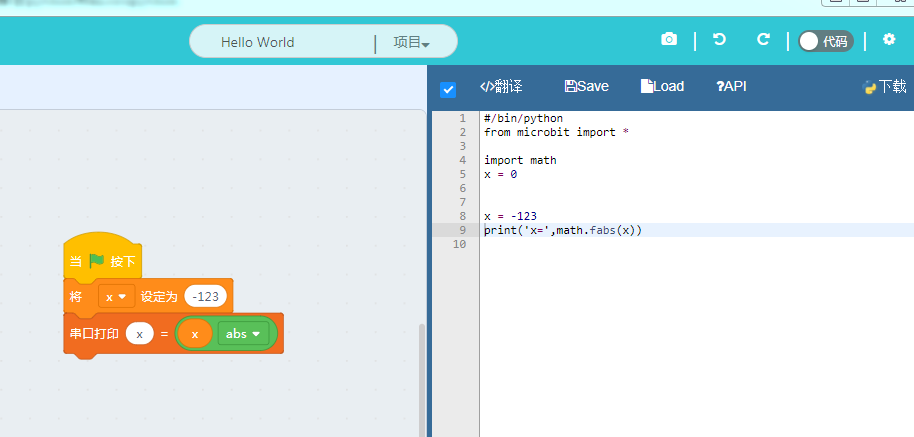# Python基础篇2¶

## python的import方法¶

microbit的每一个程序我们总能看到类似的`from microbit import *`或者`import microbit`,两者的区别是什么呢？那么如何正确使用呢？import进来的东西都到哪里去了呢？

```import microbit
print(dir(microbit))
print(locals())
```

``` ['__name__', 'Image', 'display', 'button_a', 'button_b', 'accelerometer', 'compass', 'i2c', 'uart', 'spi', 'reset', 'sleep', 'running_time', 'panic', 'temperature', 'pin0', 'pin1', 'pin2', 'pin3', 'pin4', 'pin5', 'pin6', 'pin7', 'pin8', 'pin9', 'pin10', 'pin11', 'pin12', 'pin13', 'pin14', 'pin15', 'pin16', 'pin19', 'pin20']

{'__name__': '__main__', 'microbit': <module 'microbit'>}
```

```from microbit import *

print(locals())
```

``` {'pin15': <MicroBitDigitalPin>, 'pin2': <MicroBitTouchPin>, 'pin0': <MicroBitTouchPin>, 'pin1': <MicroBitTouchPin>, 'pin3': <MicroBitAnalogDigitalPin>, 'pin6': <MicroBitDigitalPin>, 'pin4': <MicroBitAnalogDigitalPin>, 'i2c': <MicroBitI2C>, 'pin5': <MicroBitDigitalPin>, 'pin7': <MicroBitDigitalPin>, 'pin8': <MicroBitDigitalPin>, '__name__': '__main__', 'Image': <class 'MicroBitImage'>, 'pin9': <MicroBitDigitalPin>, 'pin14': <MicroBitDigitalPin>, 'pin16': <MicroBitDigitalPin>, 'reset': <function>, 'pin19': <MicroBitDigitalPin>, 'temperature': <function>, 'sleep': <function>, 'pin20': <MicroBitDigitalPin>, 'button_a': <MicroBitButton>, 'button_b': <MicroBitButton>, 'running_time': <function>, 'accelerometer': <MicroBitAccelerometer>, 'display': <MicroBitDisplay>, 'uart': <MicroBitUART>, 'spi': <MicroBitSPI>, 'panic': <function>, 'pin13': <MicroBitDigitalPin>, 'pin12': <MicroBitDigitalPin>, 'pin11': <MicroBitDigitalPin>, 'pin10': <MicroBitAnalogDigitalPin>, 'compass': <MicroBitCompass>}
```

```import microbit
microbit.display.show(microbit.Image.HEART)
```

```from microbit import *
display.show(Image.HEART)
```

```from microbit import display,Image
display.show(Image.HEART)
```

## python的数组(列表)类型¶

```a = [1, 2, 3, 4, 5]
print(a)
print(type(a))
print(dir(a))
```

```<class 'list'>

['append', 'clear', 'copy', 'count', 'extend', 'index', 'insert', 'pop', 'remove', 'reverse', 'sort']
```

```a = [1, 2, 3, 4, 5]
print(a)
a.append(6)
print(a)
a.remove(3)
print(a)
a.pop(0)
print(a)
```

```a = [1, 2, 3, 4, 5]
print(a)
print(len(a))
```

```from microbit import *

boat1 = Image("05050:"
"05050:"
"05050:"
"99999:"
"09990")

boat2 = Image("00000:"
"05050:"
"05050:"
"05050:"
"99999")

boat3 = Image("00000:"
"00000:"
"05050:"
"05050:"
"05050")

boat4 = Image("00000:"
"00000:"
"00000:"
"05050:"
"05050")

boat5 = Image("00000:"
"00000:"
"00000:"
"00000:"
"05050")

boat6 = Image("00000:"
"00000:"
"00000:"
"00000:"
"00000")

all_boats = [boat1, boat2, boat3, boat4, boat5, boat6]
```

```display.show(all_boats)
```

```from microbit import *
clock = list(Image.ALL_CLOCKS)
print(clock)
display.show(clock)
```

```[Image('00900:00900:00900:00000:00000:'),
Image('00090:00090:00900:00000:00000:'),
Image('00000:00099:00900:00000:00000:'),
Image('00000:00000:00999:00000:00000:'),
Image('00000:00000:00900:00099:00000:'),
Image('00000:00000:00900:00090:00090:'),
Image('00000:00000:00900:00900:00900:'),
Image('00000:00000:00900:09000:09000:'),
Image('00000:00000:00900:99000:00000:'),
Image('00000:00000:99900:00000:00000:'),
Image('00000:99000:00900:00000:00000:'),
Image('09000:09000:00900:00000:00000:')]
```

## python的字典类型¶

```simpledict = {'a': 'apple', 'b': 'banana', 'c': 'cat', 'd': 'dog'};
print(simpledict)
print(type(simpledict))
print(dir(simpledict))
```

```{'a': 'apple', 'd': 'dog', 'c': 'cat', 'b': 'banana'}

<class 'dict'>

['clear', 'copy', 'fromkeys', 'get', 'items', 'keys', 'pop', 'popitem', 'setdefault', 'update', 'values', '__getitem__', '__setitem__', '__delitem__']
```

```simpledict = {'a': 'apple', 'b': 'banana', 'c': 'cat', 'd': 'dog'};

simpledict.update({'x': 123})
simpledict.update({'y': 0.123})

print(simpledict)
```

```simpledict = {'a': 'apple', 'b': 'banana', 'c': 'cat', 'd': 'dog'};

simpledict['x'] = 123
simpledict['y'] = 0.123

print(simpledict)
```

```simpledict = {'a': 'apple', 'b': 'banana', 'c': 'cat', 'd': 'dog'};
del simpledict['a']
print(simpledict)
```

## python的其他数学函数¶